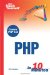# Multidimensional Arrays

It is possibleand often very usefulto use arrays to store two-dimensional or even multidimensional data.

#### Accessing Two-Dimensional Data

In fact, a two-dimensional array is an array of arrays. Suppose you were to use an array to store the average monthly temperature, by year, using two key dimensionsthe month and the year. You might display the average temperature from February 1995 as follows:

` echo \$temps["feb"]; `

Because \$temps is an array of arrays, \$temps is an array of temperatures, indexed by month, and you can reference its elements by adding the key name in square brackets.

#### Defining a Multidimensional Array

Defining a multidimensional array is fairly straightforward, as long as you remember that what you are working with is actually an array that contains more arrays.

You can initialize values by using references to the individual elements, as follows:

` \$temps["feb"] = 41; `

You can also define multidimensional arrays by nesting the array function in the appropriate places. The following example defines the first few months for three years (the full array would clearly be much larger than this):

` \$temps = array (     1995 => array ("jan" => 36, "feb" => 42, "mar" => 51),     1996 => array ("jan" => 37, "feb" => 42, "mar" => 49),     1997 => array ("jan" => 34, "feb" => 40, "mar" => 50) ); `

The print_r function can follow as many dimensions as an array contains, and the formatted output will be indented to make each level of the hierarchy readable. The following is the output from the three-dimensional \$temps array just defined:

` Array (      => Array         (             [jan] => 36             [feb] => 42             [mar] => 51         )      => Array         (             [jan] => 37             [feb] => 42             [mar] => 49         )      => Array         (             [jan] => 34             [feb] => 40             [mar] => 50         ) ) `Sams Teach Yourself PHP in 10 Minutes
ISBN: 0672327627
EAN: 2147483647
Year: 2005
Pages: 151
Authors: Chris Newman

Similar book on Amazon